Anti-aliasing in computer graphics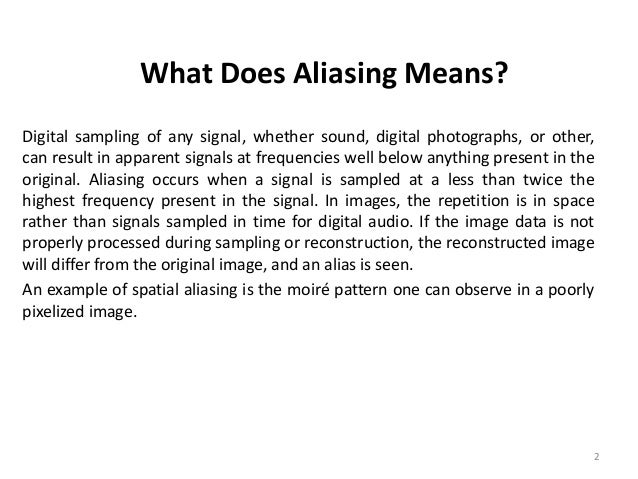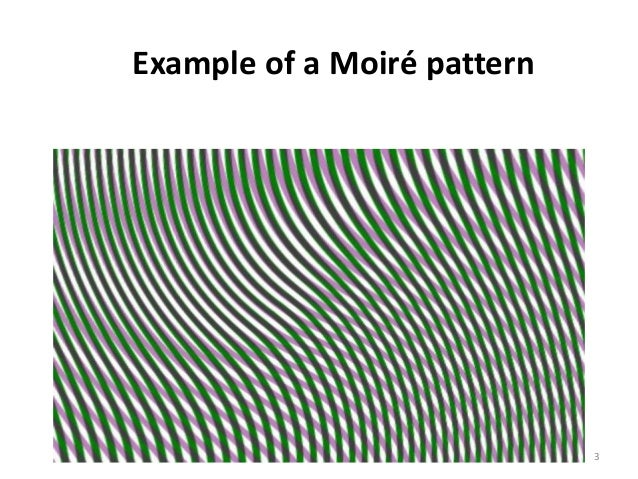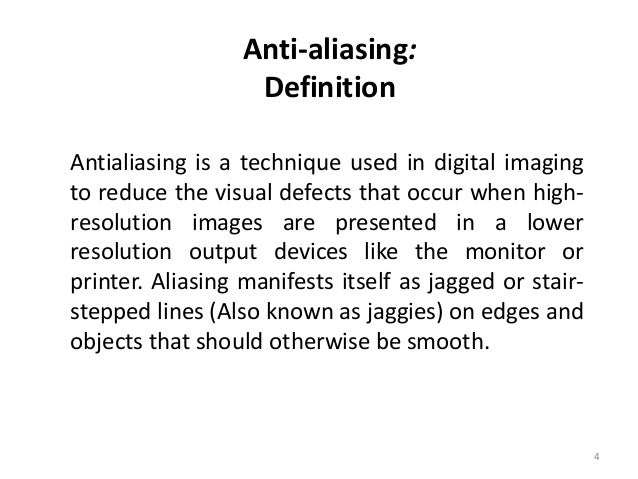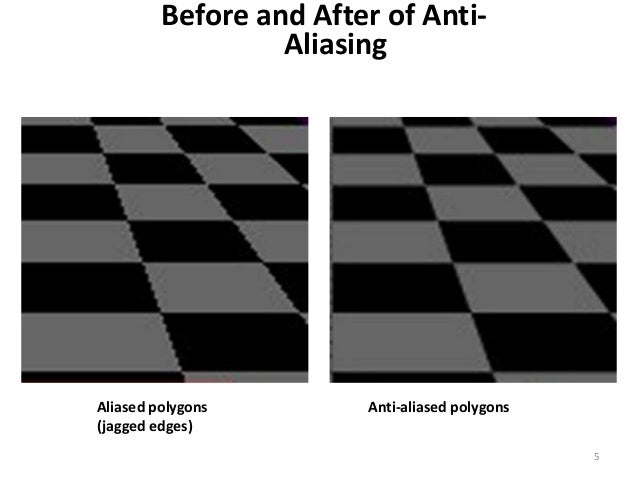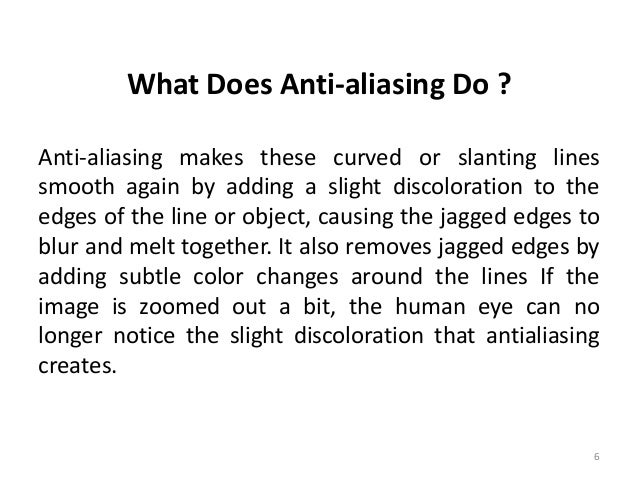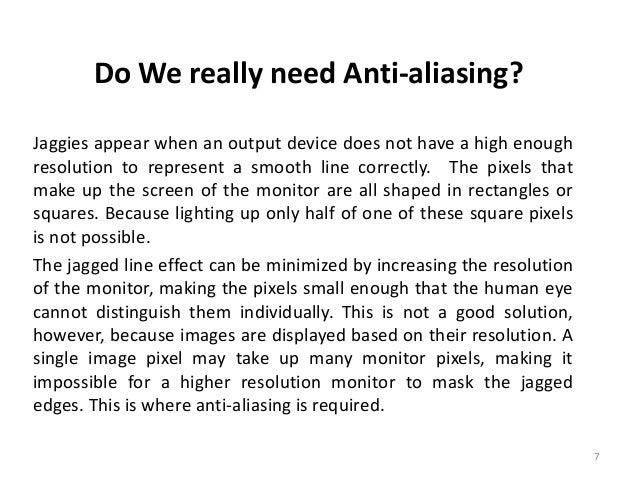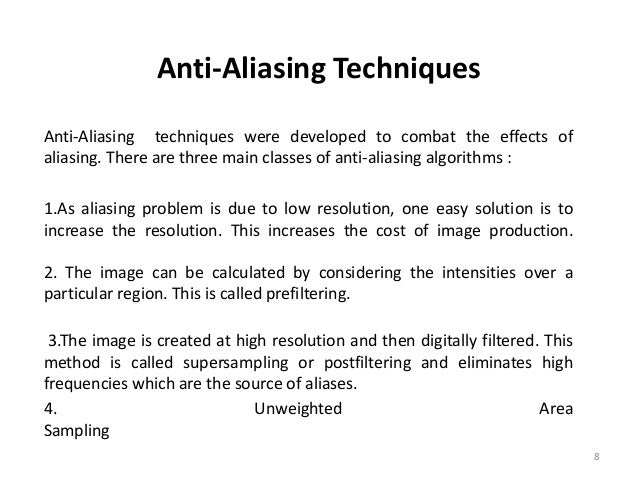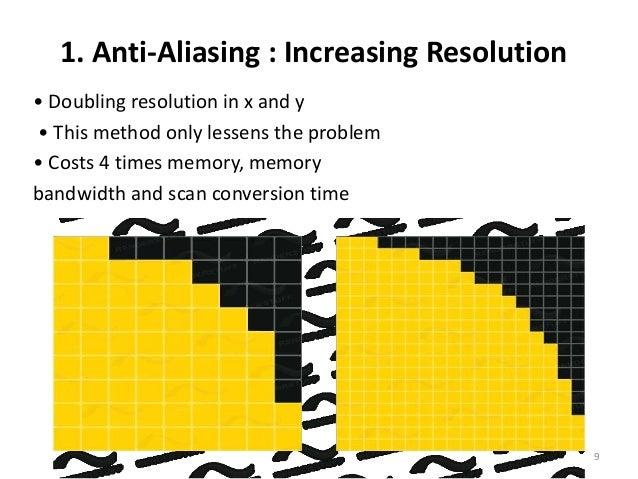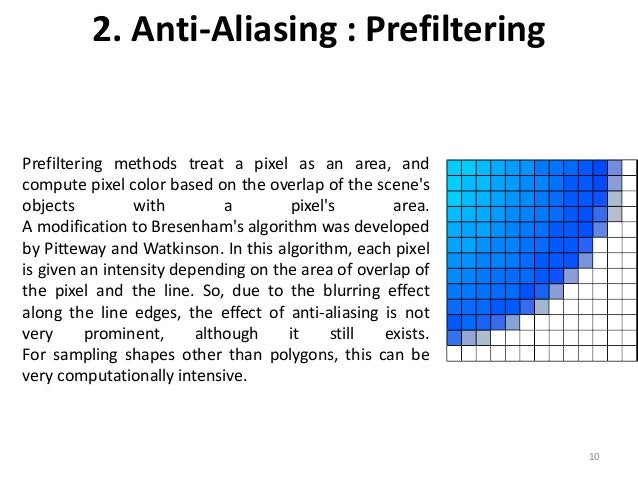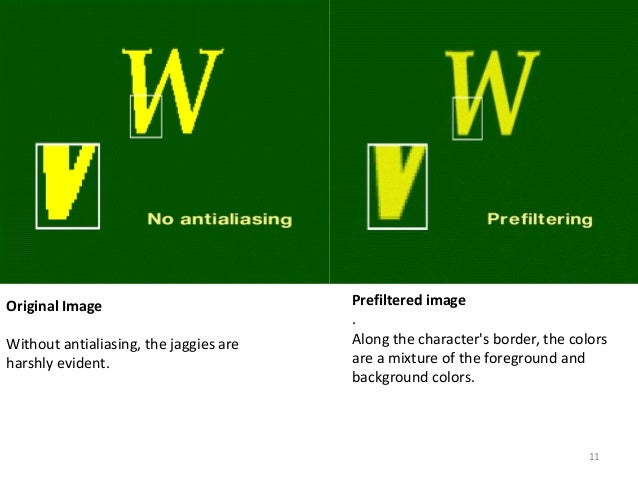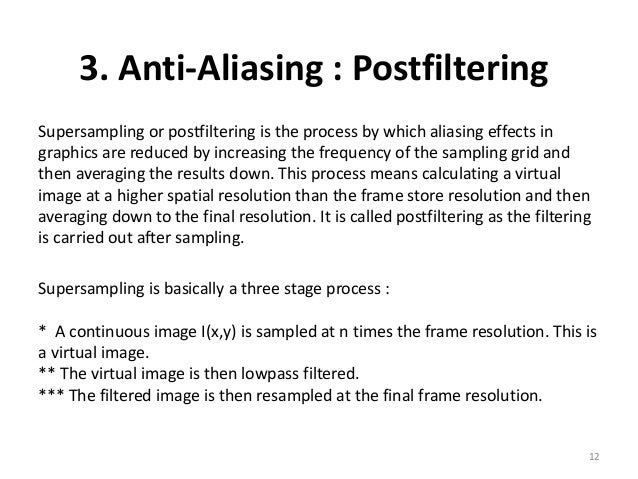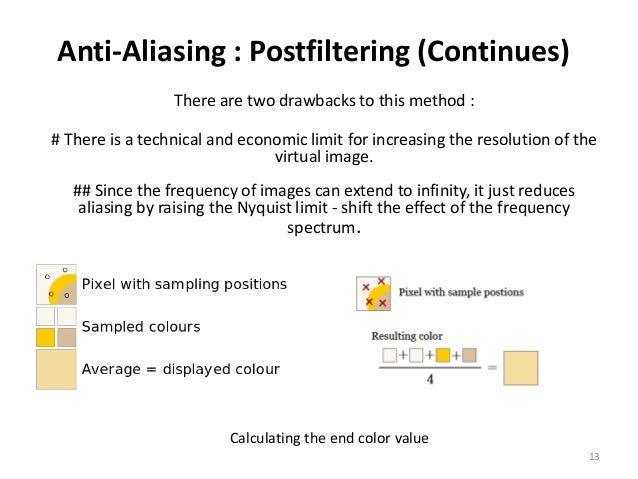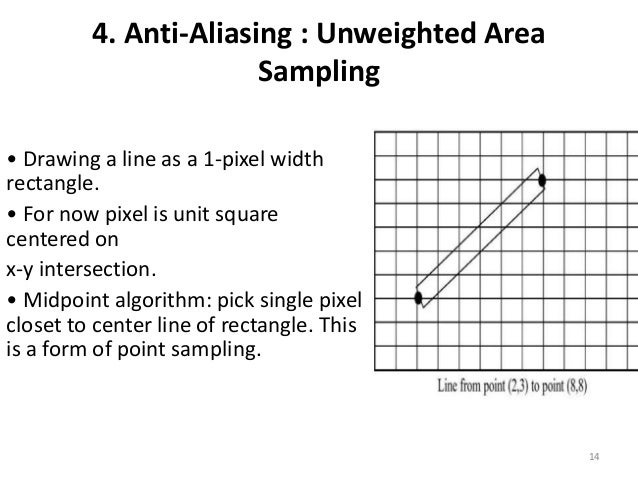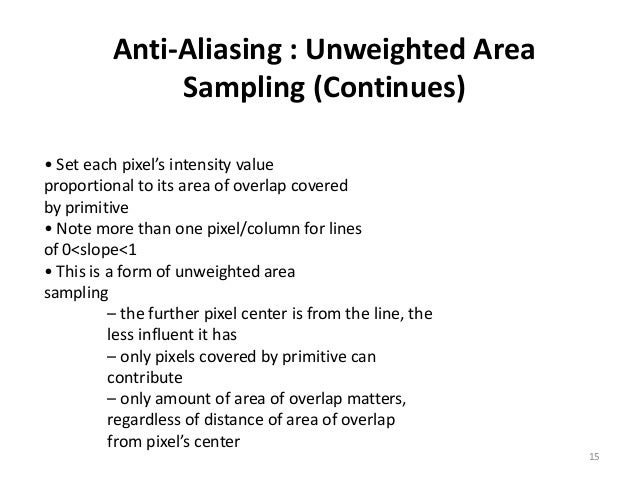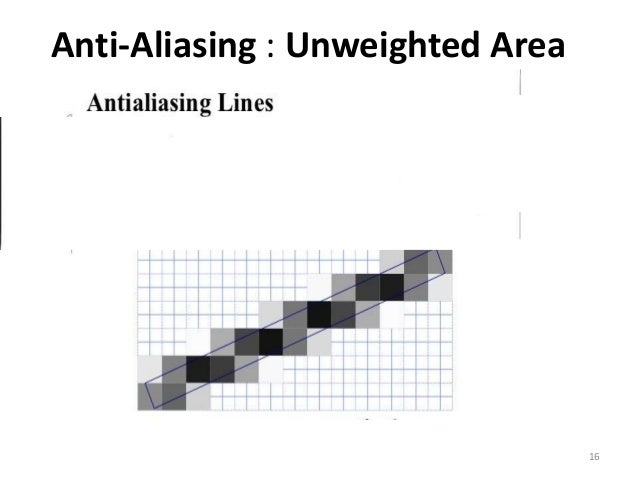Anti-Aliasing

• Raster algorithms generate jagged edges
• Discrete sampling of continuous function
• Undersampling (low frequency sampling) causes aliasing

Nyquest sampling frequency ( f)

f= 2 fmax

n = c / l = (nm/sec)/(nm/cycle) = cycles/sec

c = nm / sec = speed of light

n (wavelength/second)

l = nm / wavelength

cycle = wavelength

and

Dxsample Dxcycles / 2

Nyquest sampling interval for Dxsample  interval

where Dxcycles = 1 / fmax     or       fmax =  1 / Dxcycles

as above          n a 1 / l

• Raster graphics increases resolution but
• Frame buffer has limits
• Require arbitrary resolution

Super sampling => for images

• increase sampling rate by treating screen as if covered with finer grid
• use multiple sampling points across finer grid to determine appropriate intensity level for each pixel

Lines =>

• Calculate area of overlap for each pixel => area sampling

Super sampling straight lines

Solutions

1. For greyscale display of straight line:
• Divide each pixel into subpixels
• Count number of pixels along line path
• Set pixel intensity to subpixel count

o      e.g. divide each pixel in the 3 x 3 = 9 subpixels

o      use Bresenham’s algorithm to determine coverage

o      Four intensity levels – including black

• all 3pixels on line
• 2 pixels on line
• 1 pixel on line
• 0 pixel on line
1. Finite size line width

Super sample

• Set each pixel intensity proportional to number of pixels inside polygon representing line area
• Subpixel in line if LLC of pixel is inside polygon boundary

o      Adv. => number of available intensities = total number of pixels in area

o      Adv. => total line intensity distributed over more pixels

o      Adv.=> can blend colors

• e.g. 5 pixels in red, 4 in blue
• Pixel color = (5 * red + 4 * blue)/9

• Super sampling gives weight to interior pixels

e.g.

 2 1 2 1 1 2 4 2 0 1 2 1 0 1 2

Sum of matrix element values = 16

Weight = value / sum

e.g.    at (1,1) weight = 4 / 16/ = ¼

at (0,0) weight = 1 /16

• Can extend masks over adjacent pixels